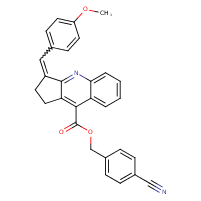Structure InformationCompound Identification

SMILES

COC1=CC=C(C=C2CCC3=C(C(=O)OCC4=CC=C(C=C4)C#N)C4=CC=CC=C4N=C23)C=C1

InChIKey

InChIKey=VTYCQWUOHOZJMA-UHFFFAOYSA-N

Formula

C29H22N2O3

Mass

446.506